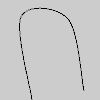# curve_vertex()#

Specifies vertex coordinates for curves.

## Examples#def setup():
py5.no_fill()
py5.begin_shape()
py5.curve_vertex(84, 91)
py5.curve_vertex(84, 91)
py5.curve_vertex(68, 19)
py5.curve_vertex(21, 17)
py5.curve_vertex(32, 100)
py5.curve_vertex(32, 100)
py5.end_shape()


## Description#

Specifies vertex coordinates for curves. This method may only be used between begin_shape() and end_shape() and only when there is no MODE parameter specified to begin_shape(). The first and last points in a series of curve_vertex() lines will be used to guide the beginning and end of the curve. A minimum of four points is required to draw a tiny curve between the second and third points. Adding a fifth point with curve_vertex() will draw the curve between the second, third, and fourth points. The curve_vertex() method is an implementation of Catmull-Rom splines. Using the 3D version requires rendering with P3D.

Underlying Processing method: curveVertex

## Signatures#

curve_vertex(
x: float,  # the x-coordinate of the vertex
y: float,  # the y-coordinate of the vertex
/,
) -> None

curve_vertex(
x: float,  # the x-coordinate of the vertex
y: float,  # the y-coordinate of the vertex
z: float,  # the z-coordinate of the vertex
/,
) -> None


Updated on March 06, 2023 02:49:26am UTC